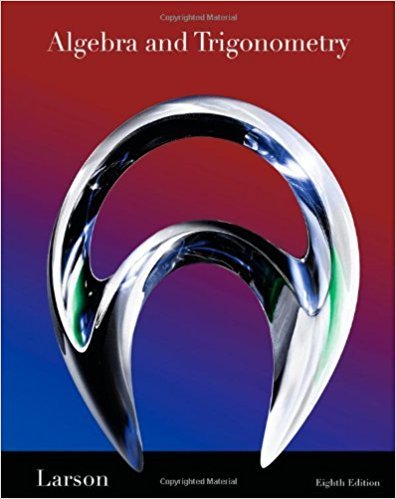×
×

# Solutions for Chapter 10.4: The Determinant of a Square Matrix## Full solutions for Algebra and Trigonometry | 8th Edition

ISBN: 9781439048474Solutions for Chapter 10.4: The Determinant of a Square Matrix

Solutions for Chapter 10.4
4 5 0 300 Reviews
21
1
##### ISBN: 9781439048474

Since 106 problems in chapter 10.4: The Determinant of a Square Matrix have been answered, more than 49404 students have viewed full step-by-step solutions from this chapter. This expansive textbook survival guide covers the following chapters and their solutions. This textbook survival guide was created for the textbook: Algebra and Trigonometry, edition: 8. Algebra and Trigonometry was written by and is associated to the ISBN: 9781439048474. Chapter 10.4: The Determinant of a Square Matrix includes 106 full step-by-step solutions.

Key Math Terms and definitions covered in this textbook
• Back substitution.

Upper triangular systems are solved in reverse order Xn to Xl.

• Cofactor Cij.

Remove row i and column j; multiply the determinant by (-I)i + j •

• Cyclic shift

S. Permutation with S21 = 1, S32 = 1, ... , finally SIn = 1. Its eigenvalues are the nth roots e2lrik/n of 1; eigenvectors are columns of the Fourier matrix F.

• Determinant IAI = det(A).

Defined by det I = 1, sign reversal for row exchange, and linearity in each row. Then IAI = 0 when A is singular. Also IABI = IAIIBI and

• Diagonal matrix D.

dij = 0 if i #- j. Block-diagonal: zero outside square blocks Du.

• Ellipse (or ellipsoid) x T Ax = 1.

A must be positive definite; the axes of the ellipse are eigenvectors of A, with lengths 1/.JI. (For IIx II = 1 the vectors y = Ax lie on the ellipse IIA-1 yll2 = Y T(AAT)-1 Y = 1 displayed by eigshow; axis lengths ad

• Fibonacci numbers

0,1,1,2,3,5, ... satisfy Fn = Fn-l + Fn- 2 = (A7 -A~)I()q -A2). Growth rate Al = (1 + .J5) 12 is the largest eigenvalue of the Fibonacci matrix [ } A].

• Free variable Xi.

Column i has no pivot in elimination. We can give the n - r free variables any values, then Ax = b determines the r pivot variables (if solvable!).

• Hessenberg matrix H.

Triangular matrix with one extra nonzero adjacent diagonal.

• Hypercube matrix pl.

Row n + 1 counts corners, edges, faces, ... of a cube in Rn.

• Linear combination cv + d w or L C jV j.

• Normal matrix.

If N NT = NT N, then N has orthonormal (complex) eigenvectors.

• Orthogonal matrix Q.

Square matrix with orthonormal columns, so QT = Q-l. Preserves length and angles, IIQxll = IIxll and (QX)T(Qy) = xTy. AlllAI = 1, with orthogonal eigenvectors. Examples: Rotation, reflection, permutation.

• Orthogonal subspaces.

Every v in V is orthogonal to every w in W.

• Particular solution x p.

Any solution to Ax = b; often x p has free variables = o.

• Pascal matrix

Ps = pascal(n) = the symmetric matrix with binomial entries (i1~;2). Ps = PL Pu all contain Pascal's triangle with det = 1 (see Pascal in the index).

• Plane (or hyperplane) in Rn.

Vectors x with aT x = O. Plane is perpendicular to a =1= O.

• Random matrix rand(n) or randn(n).

MATLAB creates a matrix with random entries, uniformly distributed on [0 1] for rand and standard normal distribution for randn.

• Special solutions to As = O.

One free variable is Si = 1, other free variables = o.

• Symmetric factorizations A = LDLT and A = QAQT.

Signs in A = signs in D.

×